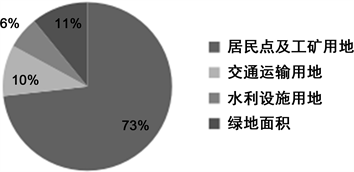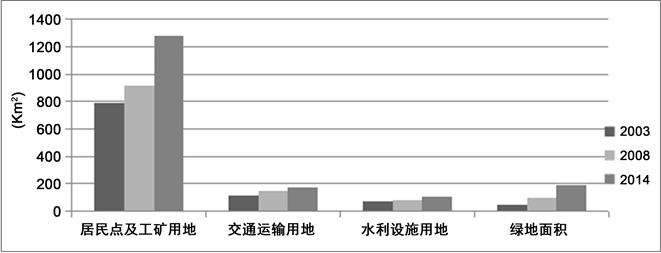昆明市城市用地结构对空间绩效的影响研究The Impact of Urban Land Use Structure on Spatial Performance in Kunming

• 全文下载: PDF(962KB)    PP.120-129   DOI: 10.12677/ULU.2018.64015
• 下载量: 163  浏览量: 341   国家自然科学基金支持

The principle of information entropy and equilibrium degree of the city based on land use in Kunming City, 2003, 2008 and 2014 were analyzed and calculated with the change of city structure, constructing the index system of Kunming city spatial performance, calculating the index weight according to the comprehensive evaluation model to calculate the spatial performance value. AHP decision analyzes the structure and performance of Kunming with information entropy value of linear regression analysis of SPSS software. The results show that the urban land use structure of Kunming is constantly improving and tends to be balanced during the study period. With the change of urban land use structure, the urban spatial performance value has been improved correspondingly, which indicates that the information entropy of urban land structure has a certain pulling effect on the spatial performance.

1. 引言

2. 城市土地利用结构的计量方法

2.1. 数据来源

2.2. 城市土地利用结构计算方法

2.2.1. 城市土地利用结构的信息熵

$S=\underset{i=1}{\overset{n}{\sum }}\text{ }{S}_{i}$

$\underset{i=1}{\overset{n}{\sum }}\text{ }{P}_{i}=1$

$H=-\underset{i=1}{\overset{n}{\sum }}\text{ }{P}_{i}\mathrm{ln}\left(Pi\right)$

0，当整地区各类职能土地面积所占比例相等，即  ，信息熵则为最大值ln(n)。由此可知，城市土地类型越多且各职能土地面积相差越小，信息熵值越大。

2.2.2. 城市土地利用结构的均衡度和优势度

$E=\frac{-{\sum }_{i=1}^{n}{P}_{i}\mathrm{ln}\left({P}_{i}\right)}{\mathrm{ln}\left(n\right)}$

$D=1-E$

3. 昆明城市土地利用结构分析

3.1. 城市土地利用结构及变化趋势分析

2014年，昆明市的城区面积为2295 km2，建成区面积为524.62 km2，根据数据和城市建设用地划分依据现将城市用地划分为4类：居民及工矿用地，交通运输用地，水利设施用地和绿地。其中居民及工矿用地1275 km2，交通运输用地171 km2，水利设施用地107 km2，绿地面积190 km2。各类职能用地占建设用地面积的比分别为73.19%，9.82%，6.14%，10.85% (图1)。Figure 1. The proportion of land use structure in Kunming in 2014Figure 2. Changes of land use structure in Kunming over the years

3.2. 城市土地利用结构信息熵的计算及分析Table 1. Urban land use structure, scale and information entropy, equilibrium and dominance in Kunming

4 城市空间绩效评价指标体系的建立及计算

4.1. 城市空间绩效评价指标的建立Table 2. Index system of urban spatial performance evaluation

4.2. 评级指标权重的确定Table 3. Final weight value of urban spatial performance evaluation index

4.3. 评价指标标准化处理

${{x}^{\prime }}_{ij}=\frac{{x}_{ij}}{\text{max}\left\{{x}_{ij}\right\}},\text{\hspace{0.17em}}\text{\hspace{0.17em}}\text{\hspace{0.17em}}i=1,2,\cdots ,m;j=1,2,\cdots ,n$

4.4. 空间绩效的综合评价指标计算

$A={\sum }_{i=1}^{n}{A}_{i}×{W}_{i},\text{\hspace{0.17em}}\text{\hspace{0.17em}}\text{\hspace{0.17em}}i=1,2,\cdots ,n$Table 4. Urban spatial performance evaluation index of Kunming

5. 土地利用结构与空间绩效的相关分析

5.1. 城市用地规模与空间绩效相关性分析

${r}_{xy}=\frac{{\sum }_{i=1}^{n}\left({x}_{i}-\stackrel{¯}{x}\right)\left({y}_{i}-\stackrel{¯}{y}\right)}{\sqrt{{\sum }_{i=1}^{n}{\left({x}_{i}-\stackrel{¯}{x}\right)}^{2}}\sqrt{{\sum }_{i=1}^{n}{\left({y}_{i}-\stackrel{¯}{y}\right)}^{2}}}$Table 5. Correlation between land use scale and performance in Kunming

5.2. 结构信息熵与空间绩效线性的回归分析

${Y}_{i}=\alpha X+\beta$

5.2.1. 信息熵与经济绩效的关系

${Y}_{1}=3.805X-2.952$ ${F}_{1}=24.237$ ${R}_{1}=0.98$

5.2.2. 信息熵与社会结构绩效的关系

${Y}_{2}=1.105X-0.704$ ${F}_{2}=22.110$ ${R}_{2}=0.978$

5.2.3. 信息熵与资源环境绩效的关系

${Y}_{3}=0.874X-0.604$ ${F}_{3}=31.409$ ${R}_{3}=0.984$

5.2.4. 信息熵与空间绩效的关系

${Y}_{4}=-0.34X+0.419$ ${F}_{4}=0.173$ ${R}_{4}=-0.384$

5.2.5. 信息熵与综合绩效的关系

${Y}_{5}=5.444X-3.841$ ${F}_{5}=7.548$ ${R}_{5}=0.940$

6. 结论与讨论

6.1. 结论

1) 研究期间内昆明城市建设用地面积和各二级分类土地面积都在扩大，绿地面积在总建设用地面积的比例在逐年增大，但其余职能用地类型用地比例在减小。依据信息熵原理算出的信息熵在这3年里分别为0.7939，0.8484和0.8686，其数值在逐渐增大，表明城市土地利用结构朝均衡化趋势发展。

2) 经过分析可以看出三年里综合空间绩效值最高的为2008年，2014年位居其次，2003年排在最末；研究期间内间昆明市的经济绩效值、社会结构绩效值和资源环境绩效值都呈现逐年递增的情况，而空间结构绩效值则为2008年最高，为0.165；2003年排名随后，为0.140；2014年空间结构绩效值最低，为0.098；究其原因是人口增长率和建成区面积增长率在2008年增长最大，而2014年变化最小。综合绩效值则是2008年最高，2014年排名第二，2003年数值最低，说明对于综合绩效而言，其空间发展规划和城市扩展方式对综合绩效值存在较大影响。

3) 昆明城市土地利用结构信息熵的变化对经济绩效和资源环境绩效存在影响。对回归方程和相关系数进行分析检验得出：昆明城市土地利用结构信息熵与经济绩效和资源环境绩效有显著地正相关关系。当结构信息熵改变时，经济绩效会有3.805倍的增长，资源环境绩效呈0.874倍增长。

6.2. 讨论

NOTES

*通讯作者。

  周丹丹. 城市土地利用绩效评价研究[D]: [硕士学位论文]. 重庆: 重庆大学, 2010.  王万茂, 董祚继, 等. 土地利用规划学(第一版) [M]. 北京: 科学出版社, 2006: 142.  United Nations (1996) Indicators of Sustainable Development: Frame-work and Methodologies. United Nations, New York.  Armstrong, M. and Baron, A. (1998) Performance Management. The Cromwell Press, London.  彭坤焘, 赵民. 关于“城市空间绩效”及城市规划的作为[J]. 城市归划, 2010, 34(8): 9-17.  颜文涛, 萧敬豪, 胡海, 邹锦. 城市空间结构的环境绩效:进展与思考[J]. 城市规划学刊, 2012(5): 50-59.  刁星, 程文. 城市空间绩效评价指标体系构建及实践[J]. 规划师, 2015, 31(8): 110-115.  李江, 郭庆胜. 基于信息熵的城市用地结构动态演变分析[J]. 长江流域资源与环境, 2002, 11(5): 393-397.  欧聪, 张坤. 城市土地利用结构演变分析及其驱动机制研究以长沙市为例[J]. 上海国土资源, 2015, 36(3): 39-43.  刘学, 孙泰森. 太原城市土地利用结构演变及驱动力分析[J]. 山西师范大学学报(自然科学版), 2014, 28(4): 84-89.  马菊. 陕西省中小城市空间绩效评价指标体系构建研究[D]: [硕士学位论文]. 西安: 西安建筑科技大学, 2014.  张培培. 基于空间绩效评价的焦作市空间发展模式比较研究[D]: [硕士学位论文]. 西安: 西安建筑科技大学, 2014.  吴一洲, 吴次芳, 罗文斌, 王琳. 浙江省城市土地利用绩效的空间格局及其机理研究[J]. 中国土地科学, 2009(10): 41-46.  徐建华. 计量地理学(第二版) [M]. 北京: 高等教育出版社, 2014: 84.  张冰洁. 哈尔滨市城市土地利用结构变化与效益关系研究[D]: [硕士学位论文]. 哈尔滨: 东北农业大学, 2013.